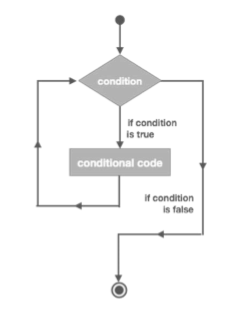# Jython - Loops

In general, statements in a program are executed sequentially: The first statement in a function is executed first, followed by the second, and so on. There may be a situation when you need to execute a block of code several number of times. Statements that provide such repetition capability are called looping statements.

In Jython, a loop can be formed by two statements, which are −

• The while statement and

• The for statement

## The WHILE Loop

A while loop statement in Jython is similar to that in Java. It repeatedly executes a block of statements as long as a given condition is true. The following flowchart describes the behavior of a while loop.The general syntax of the while statement is given below.

```while expression:
statement(s)
```

The following Jython code uses the while loop to repeatedly increment and print value of a variable until it is less than zero.

```count = 0
while count<10:
count = count+1
print "count = ",count
print "Good Bye!"
```

Output − The output would be as follows.

```count =  1
count =  2
count =  3
count =  4
count =  5
count =  6
count =  7
count =  8
count =  9
count =  10
Good Bye!
```

## The FOR Loop

The FOR loop in Jython is not a counted loop as in Java. Instead, it has the ability to traverse elements in a sequence data type such as string, list or tuple. The general syntax of the FOR statement in Jython is as shown below −

```for iterating_var in sequence:
statements(s)
```

We can display each character in a string, as well as each item in a List or Tuple by using the FOR statement as shown below −

```#each letter in string
for letter in 'Python':
print 'Current Letter :', letter
```

Output − The output would be as follows.

```Current Letter : P
Current Letter : y
Current Letter : t
Current Letter : h
Current Letter : o
Current Letter : n
```

Let us consider another instance as follows.

```#each item in list
libs = [‘PyQt’, 'WxPython',  'Tkinter']
for lib in libs:        # Second Example
print 'Current library :', lib
```

Output − The output will be as follows.

```Current library : PyQt
Current library : WxPython
Current library : Tkinter
```

Here is another instance to consider.

```#each item in tuple
libs = (‘PyQt’, 'WxPython',  'Tkinter')
for lib in libs:        # Second Example
print 'Current library :', lib
```

Output − The output of the above program is as follows.

```Current library : PyQt
Current library : WxPython
Current library : Tkinter
```

In Jython, the for statement is also used to iterate over a list of numbers generated by range() function. The range() function takes following form −

```range[([start],stop,[step])
```

The start and step parameters are 0 and 1 by default. The last number generated is stop step. The FOR statement traverses the list formed by the range() function. For example −

```for num in range(5):
print num
```

It produces the following output −

```0
1
2
3
4
```